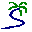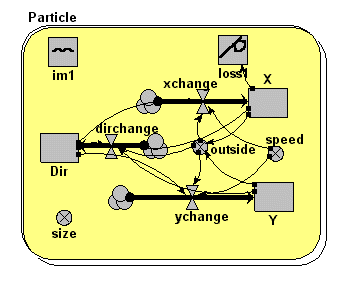# Model entry

Lagrangian particle movement - Model catalogue - Simulistics.com

 Search Simulistics Model catalogue Listed by keyword Listed by ID Listed by title Listed by date added

# Lagrangian particle movement

Model : lagrangian1
Simile version : 3.1+
Keywords : Movement ; Individual-based ;

## Description

This is a (small) modification of a basic individual-based movement model. In this case, particles are being continuously created and destroyed, the random movement is rather less - particles tend to move in straight lines - and an impermeable 'barrier' has been introduced. Those particles above it are not affected, but those that would hit it change direction as they approach it and flow around it.

The original context for this model was the simulation of large numbers of CO2 molecules moving from the soil up through the forest canopy into the atmosphere above. The 'barrier' could thus represent a leaf that the moleculs need to move around. An extension of the model could then involve providing stomata that some molecules could enter, and then participate in photosynthesis.

## Files

### Model file

Click on the icon to download the model file. (You will need Simile to examine and run the model. A free evaluation version is available from the products page.)

Some browsers may attempt to display the model file, rather than open it in Simile; in this case, use the browser back button to return to this page, and use the context menu (invoked by right-clicking on the link) to save the target file to disk.lagrangian1.sml

## Diagram## Equations

```Equations in PREDATOR
Compartments:
X:    initial value=80
Dir:  initial value=rand(0,6.28)
Y:    initial value=rand(10,90)

Flows:
xchange    = if outside==1 then speed*cos(dir+3.14)else speed*cos(dir)
dirchange  = if outside==1 then 3.14/dt(1)else(if (x>30,x<50,y<70)then 0.4*(1.57-fmod(abs(dir),6.28))else 0.4*(3.14-fmod(abs(dir),6.28))+rand(-0.15,0.15)/dt(1))
ychange    = if outside==1 then speed*sin(dir+3.14)else speed*sin(dir)

Variables:
speed      = 5
size       = 1
outside    = if max(abs(50-x),abs(50-y))>50 then 1 else 0
im1        = 10
loss1      = X<0
```

## Results

|

When the model is run, and the behaviour presented using the 'lollipop' diagram, you see particles being created along the right-hand side of the area and flowing across to the left. The ones moving along the bottom then flow up and over the barrier.|